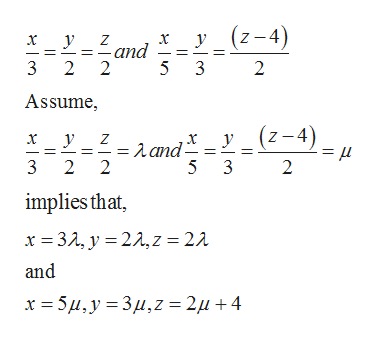In relation to vectors, lines and planes.(a) Do the lines x/3=y/2=z/2 and x/5=y/3=(z-4)/2 intersect?(b) Find equations for a line perpendicular to both of these lines.(c) What is the distance between these lines?

Question

In relation to vectors, lines and planes.

(a) Do the lines x/3=y/2=z/2 and x/5=y/3=(z-4)/2 intersect?

(b) Find equations for a line perpendicular to both of these lines.

(c) What is the distance between these lines?

Step 1

(a) Given equations of the lines are,help_outlineImage Transcriptionclose(z-4) Z -and 5 3 3 2 2 2 Assume, (z-4) Aand 3 2 2 5 3 2 implies that, x 3, 2,z 21 and x 5, y 3 2u 4 fullscreen
Step 2

Equating the coefficient of x, y, z from both equations,

Step 3

on solving 1st two equ...

Want to see the full answer?

See Solution

Want to see this answer and more?

Our solutions are written by experts, many with advanced degrees, and available 24/7

See Solution
Tagged in

Math